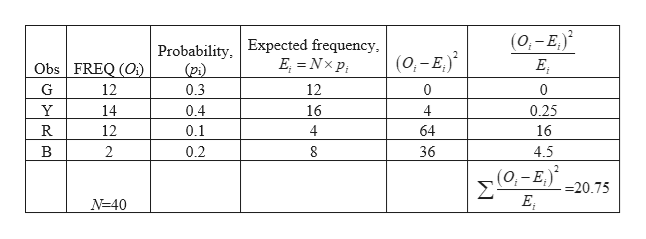# P (G) = .3 P (Y) = .4P (B) = .2P(R) = .1OBS.   G   Y  R  BFREQ. 12 14 12 2 find the test-statistics to determine this data will "FIT" the expectation

Question
5 views

P (G) = .3

P (Y) = .4

P (B) = .2

P(R) = .1

OBS.   G   Y  R  B

FREQ. 12 14 12 2

find the test-statistics to determine this data will "FIT" the expectation

check_circle

Step 1

The test statistic for determining the fit of the data is 20.75 and it is calcula...help_outlineImage Transcriptionclose(0,- E.) Expected frequency, E, = Nx p; Probability, (pi) (0,-E,) E; Obs FREQ (O) G 12 0.3 12 14 0.4 16 4 0.25 12 0.1 4 64 16 B 2 0.2 36 4.5 (0,-E,)* Σ =20.75 N=40 fullscreen

### Want to see the full answer?

See Solution

#### Want to see this answer and more?

Solutions are written by subject experts who are available 24/7. Questions are typically answered within 1 hour.*

See Solution
*Response times may vary by subject and question.
Tagged in

### Hypothesis Testing